# How to Analyze Eigenfrequencies That Change with Temperature

May 22, 2017

In some applications, particularly within the MEMS field, it is important to study the sensitivity of a device’s eigenfrequencies with respect to a variation in temperature. In this blog post, we show how to do this using COMSOL Multiphysics® version 5.3. We also explore effects like stress softening, geometric changes, and the temperature dependence of material properties.

### Studying Temperature-Dependent Eigenfrequencies

Some devices require a very high degree of frequency stability with respect to changes in the environment. The most common parameter is temperature, but the same type of phenomena could, for example, be caused by hygroscopic swelling due to changes in humidity. In very high precision applications, the frequency stability requirements might specify a precision at the ppb (parts-per-billion, 10-9) level. Setting up simulations that accurately capture such small effects can be a challenging task, since several phenomena can interact.

### Rectangular Beam Example

Consider a rectangular beam with the following data:

Property Symbol Value
Length L 10 mm
Width a 1 mm
Height b 0.5 mm
Young’s modulus E 100 GPa
Poisson’s ratio ν 0
Mass density ρ 1000 kg/m3
Coefficient of thermal expansion, x direction αx 1·10-5 1/K
Coefficient of thermal expansion, y direction αy 2·10-5 1/K
Coefficient of thermal expansion, z direction αz 3·10-5 1/K
Temperature shift ΔT 10 K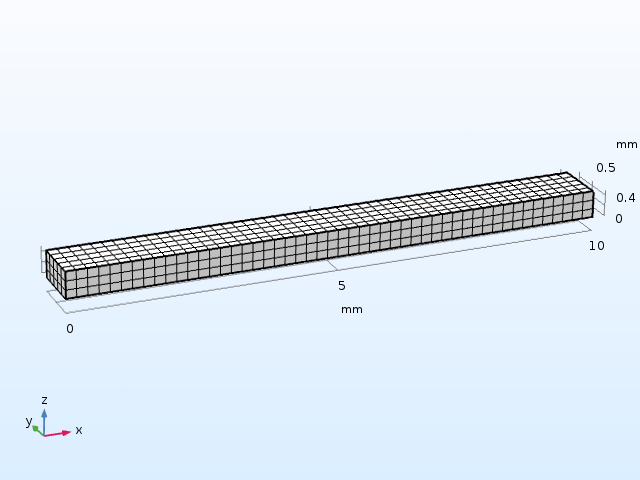The beam geometry and mesh used in the example.

The material parameters have values that are of the same order of magnitude as those for many other engineering materials. To better separate the various effects, Poisson’s ratio is set to zero, but this assumption does not change the results in any fundamental way. Orthotropic thermal expansion coefficients are used to highlight some properties of the solution.

To analyze the effect of thermal expansion, add a Prestressed Analysis, Eigenfrequency study.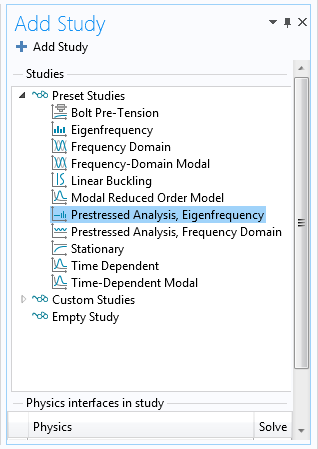Adding the Prestressed Analysis, Eigenfrequency study.

This study consists of two study steps:

1. A Stationary study step that computes the displacements and stresses caused by the thermal expansion
2. An Eigenfrequency study step in which the previously computed solution is used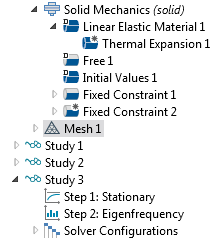The two study steps shown in the Model Builder tree.

To compute the reference solution, you either add a separate Eigenfrequency study or run the same study sequence, but without thermal expansion.

#### Eigenfrequencies of Doubly Clamped and Cantilever Beams

The eigenfrequencies of the beam have been calculated for two different types of boundary conditions:

1. A doubly clamped beam
2. A cantilever beam, where one end is fixed and the other end is free

The doubly clamped beam results are shown below.

Mode Type Eigenfrequency, Hz Eigenfrequency, Hz
ΔT = 10 K
Ratio
First bending, z direction 50713.9 50425.1 0.9943
First bending, y direction 97659.6 97526.2 0.9986
First twisting 266902 266917 1.00006
First axial 500000 500025 1.00005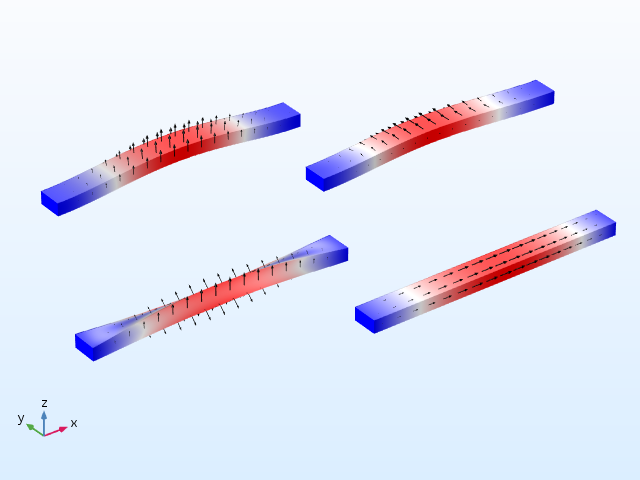Mode shapes for the doubly clamped beam.

The following table shows the cantilever beam results.

Mode Type Eigenfrequency, Hz Eigenfrequency, Hz
ΔT = 10 K
Ratio
First bending, z direction 8063.79 8066.92 1.00039
First bending, y direction 16049.1 16053.7 1.00028
First twisting 132233 132265 1.00025
First axial 250000 250050 1.0002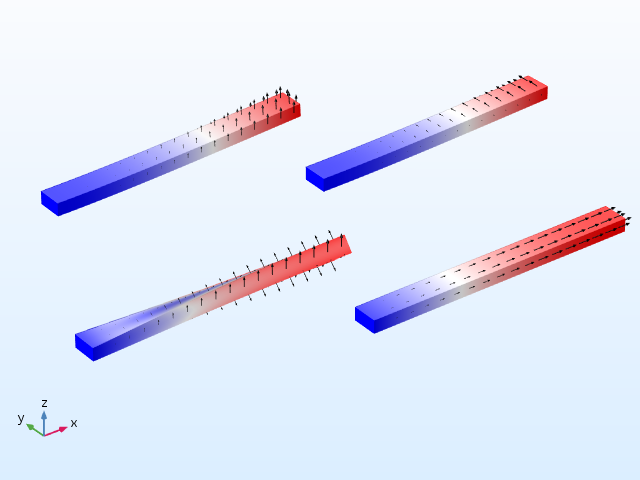Mode shapes for the cantilever beam.

The first thing to note is that the bending eigenmodes for the doubly clamped beam stand out and have a strong temperature dependence. The change is 0.6% in the first mode. For all other modes, the relative shift in frequency is significantly smaller. If you make the beam thinner, this difference would be even more pronounced. The reason for this behavior is discussed in the following sections.

### Examining the Stress-Softening Effect

In the case of the doubly clamped beam, the thermal expansion causes a compressive axial stress. With the given data, the stress is -10 MPa (computed as xΔT). This stress causes a significant reduction in the stiffness of the beam — an effect often called stress stiffening, since it typically occurs in structures with tensile stresses. However, compressive stresses soften the structure.

Another way of looking at this is by performing a linear buckling analysis. You can do so by adding a Linear Buckling study to the model and using the thermal expansion caused by ΔT = 10 K as a unit load. You will then find that the critical load factor is 80.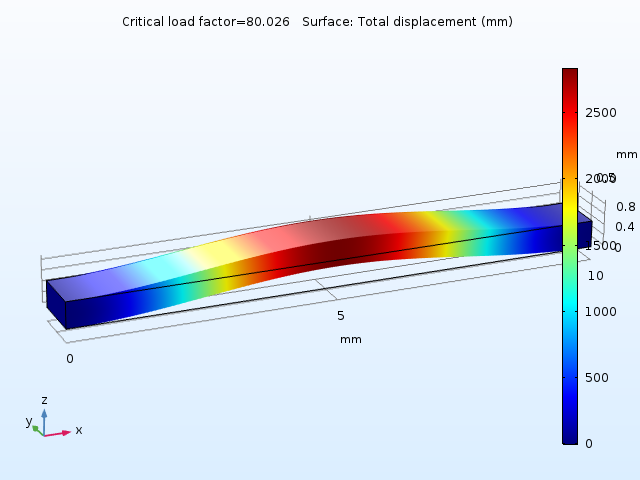The first buckling mode.

With a linear assumption, the beam becomes unstable at an 800 K temperature increase. At the buckling load, the stiffness has reached 0. Assuming that the stiffness decreases linearly with the compressive stress, the stiffness at ΔT = 10 K should be reduced by a factor of

1-\frac{1}{80} = 0.9875

Since a natural frequency is proportional to the square root of the stiffness, you can estimate the decrease to \sqrt{0.9875}=0.9937, which matches the computed value of 0.9943 well.

Stress softening also affects the twisting and axial modes, but the effect is not as obvious as it is in the bending modes.

### Evaluating How a Geometry Change Affects the Frequency

In the cantilever beam, no stresses develop when it is heated, as it simply expands. In this case, the frequency shift is due solely to the change in geometry — an effect that is much smaller than the stress-softening effect.

The natural frequencies for the bending, torsional, and axial vibration of a beam have the following dependencies on the physical properties:

f_b \propto \frac{1}{L^2} \sqrt{\frac{EI}{\rho A}}
f_t \propto \frac{1}{L} \sqrt{\frac{GK}{\rho J}}
f_a \propto \frac{1}{L} \sqrt{\frac{E}{\rho}}

Here, the following variables have been introduced:

• I = Area moment of inertia around the bending axis
• G = Shear modulus
• K = Torsional modulus
• J = Polar moment of inertia around the beam axis

It is assumed that the initial dimensions of the beam are L0 x a0 x b0, where a0 > b0. After thermal expansion, the size is L x a x b.

The expansions (strains) in the three orthogonal directions are called εx, εy, and εz; respectively. In this case, they are linearly related to the thermal expansion by εx = αxΔT, εy = αyΔT, and εz = αzΔT; but in principle, it could be any type of inelastic strain.

The geometric properties scale as:

\begin{align} L& =L_0(1+\epsilon_x)\\
A& = ab = a_0b_0(1+\epsilon_y)(1+\epsilon_z)\\
I_y& = \frac{ab^3}{12} = \frac{a_0b_0^3}{12}(1+\epsilon_y)(1+\epsilon_z)^3\\
I_z& = \frac{ba^3}{12} = \frac{b_0a_0^3}{12}(1+\epsilon_z)(1+\epsilon_y)^3\\
K& = \frac{ab^3}{3}F_1(a/b) \approx \frac{a_0b_0^3}{3}F_1(a_0/b_0)(1+\epsilon_y)(1+\epsilon_z)^3\\
J& =\frac{ab^3+ba^3}{12}=\frac{a_0b_0^3(1+\epsilon_y)(1+\epsilon_z)^3+b_0a_0^3(1+\epsilon_z)(1+\epsilon_y)^3}{12} \end{align}

The mass density also changes. Since the same mass is now confined in a larger volume,

\rho = \frac{\rho_0}{(1+\epsilon_x)(1+\epsilon_y)(1+\epsilon_z)}

By introducing these expressions into the formulas for the natural frequencies, you arrive at the following expected eigenfrequency shifts:

\frac{f_{b,z}}{f_{b0,z}} = \sqrt{\frac{(1+\epsilon_y)(1+\epsilon_z)^3}{(1+\epsilon_x)^3}} \approx 1-\frac{3\epsilon_x}{2}+\frac{\epsilon_y}{2}+\frac{3\epsilon_z}{2}
\frac{f_{b,y}}{f_{b0,y}} = \sqrt{\frac{(1+\epsilon_z)(1+\epsilon_y)^3}{(1+\epsilon_x)^3}} \approx 1-\frac{3\epsilon_x}{2}+\frac{3\epsilon_y}{2}+\frac{\epsilon_z}{2}
\frac{f_a}{f_{a0}} = \sqrt{\frac{(1+\epsilon_y)(1+\epsilon_z)}{(1+\epsilon_x)}} \approx 1-\frac{\epsilon_x}{2}+\frac{\epsilon_y}{2}+\frac{\epsilon_z}{2}

Since the thermal expansions are very small, the approximate first-order series expansions can be expected to be accurate.

For the torsional vibrations, the situation is slightly more complicated, since the powers of a and b are mixed in the expression for the polar moment J. But if you make use of the fact that a = 2b for this geometry, then it is possible to derive a similar expression.

\frac{f_{t}}{f_{t0}} = \sqrt{\frac{5(1+\epsilon_z)(1+\epsilon_y)^3}{(1+\epsilon_x)((1+\epsilon_z)^2+ 4(1+\epsilon_y)^2))}} \approx 1-\frac{\epsilon_x}{2}-\frac{3\epsilon_y}{10}+\frac{6\epsilon_z}{5}

Now, compare the computed frequency shifts with the analytical predictions for the cantilever beam. The results are shown in the table below and the agreement is very good.

Mode Type Computed Predicted
First bending, z direction 1.00039 1.00040
First bending, y direction 1.00028 1.00030
First twisting 1.00025 1.00025
First axial 1.00020 1.00020

### Analyzing the Effects of Constraint Modeling

The fixed constraints at the ends of the beam cause local stress concentrations when the temperature is increased, as the transverse displacement is constrained.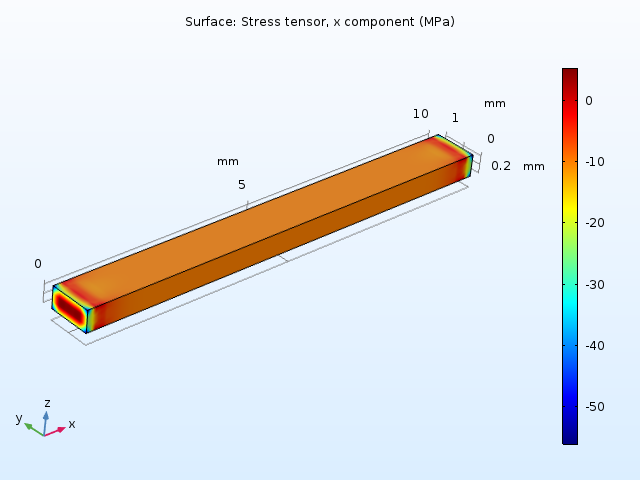The axial stress in the doubly clamped beam caused by a 10 K temperature increase.

This can have two effects:

1. Stress stiffening might be induced in a component that is expected to experience only volumetric changes
2. The cross section dimension is no longer constant, due to the restrained transverse displacement (as in the example above)

To determine what effects the constraints should have, you must rely on your engineering judgment. Usually, the component and its surroundings are subject to temperature changes. In this situation, the possibility to add a thermal expansion to constraints in COMSOL Multiphysics comes in handy. Let’s see how the solution is affected.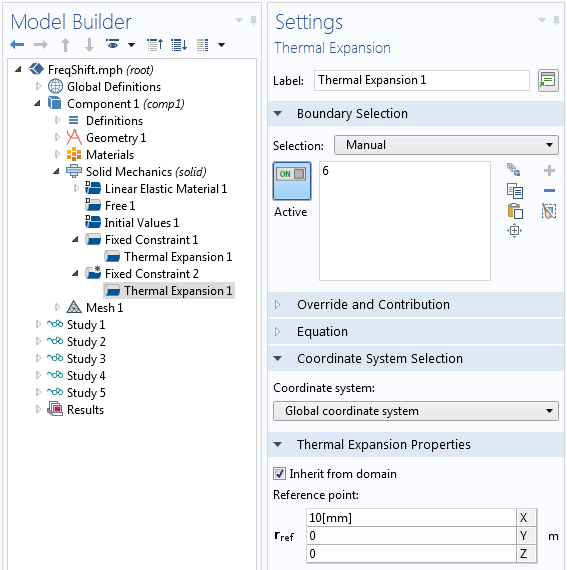Thermal expansion added to the fixed constraints for the doubly clamped beam.

For the cantilever beam, the results now change so that they perfectly match the analytical values.

Mode Type Fixed Constraints Stress-Free Constraints Predicted
First bending, z direction 1.00039 1.00040 1.00040
First bending, y direction 1.00028 1.00030 1.00030
First twisting 1.00025 1.00026 1.00025
First axial 1.00020 1.00020 1.00020

### Including Temperature Dependence in Material Data

In the analysis above, it is assumed that the material data does not depend on temperature. When looking at constrained structures (dominated by the stress-softening effect), this might be an acceptable approximation. However, with the small frequency shifts caused by geometric changes, the temperature dependence of the material must also be taken into account.

In this guide, you can see the temperature dependence of Young’s modulus for a number of metals. The stiffness decreases with temperature. For many materials, the relative change in stiffness is of an order of 10-4 K-1. This means that for a temperature change of 10 K, you can expect a relative change in material stiffness that is of the order of 0.1%. This effect might actually be larger than the geometric effect computed above.

A small note of warning: When measuring the temperature dependence of Young’s modulus, it is important to know whether or not the geometric change caused by thermal expansion has been taken into account. In other words, you must know whether the Young’s modulus is measured with respect to the original dimensions or the heated dimensions.

When it comes to mass density, the situation is easier. When performing structural mechanics analyses in COMSOL Multiphysics, the equations are formed in the material frame. Thus, the mass density should never be given an explicit temperature dependence, since that violates mass conservation.

The coefficient of thermal expansion (CTE) usually increases with temperature. The relative sensitivity is often of the order of 10-3 K-1. This sounds large, but it isn’t usually important when looking at the way the CTE enters the equations.

Most materials in the Material Library in COMSOL Multiphysics come with temperature-dependent material properties. In this example, you manually add a linear temperature dependence to the Young’s modulus with the following steps:

1. In the settings for the Basic property group, select Temperature under Model Inputs
2. Click Add to see that the variable name to be used is T
3. Write an expression for Young’s modulus that is a function of T

Alternatively, you can create a function and call it, with T as the argument.Adding a linear temperature dependence to the material.

In the settings for the Linear Elastic Material, the Model Input section is now active. You then provide a temperature to be used by the material.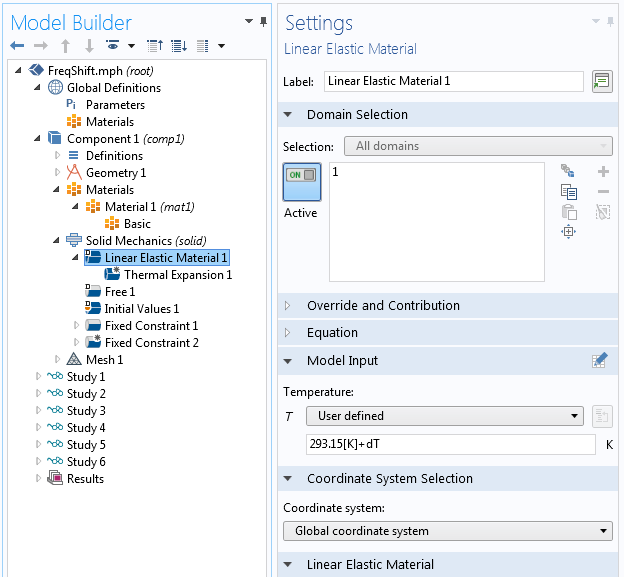Adding the temperature to the material using Model Input.

After including a reduction of Young’s modulus by 1·10-4 K-1, the resulting frequency shift turns out to be negative, rather than the positive shift observed with a constant Young’s modulus (shown in the table below).

Mode Type Stress-Free Constraints
Constant E
Stress-Free Constraints
Temperature-Dependent E
Difference
First bending, z direction 1.00040 0.99990 -0.00050
First bending, y direction 1.00030 0.99980 -0.00050
First twisting 1.00026 0.99976 -0.00050
First axial 1.00020 0.99970 -0.00050

The shift is exactly as expected for all modes — Young’s modulus is reduced by a factor 1·10-3 and the natural frequencies are proportional to its square root. Actually, you can include the change in Young’s modulus in the linearized expressions for the frequency shifts as:

\frac{f_{b,z}}{f_{b0,z}} \approx 1+ \left (-\frac{3\alpha_x}{2}+\frac{\alpha_y}{2}+\frac{3\alpha_z}{2}+\frac{\beta}{2} \right)\Delta T
\frac{f_{b,y}}{f_{b0,y}} \approx 1 + \left (-\frac{3\alpha_x}{2}+\frac{3 \alpha_y}{2}+\frac{\alpha_z}{2}+\frac{\beta}{2} \right)\Delta T
\frac{f_a}{f_{a0}} \approx 1 + \left (-\frac{\alpha_x}{2}+\frac{\alpha_y}{2}+\frac{\alpha_z}{2}+\frac{\beta}{2} \right)\Delta T
\frac{f_{t}}{f_{t0}} \approx 1 + \left (-\frac{3\alpha_x}{2}-\frac{3 \alpha_y}{10}+\frac{6\alpha_z}{5}+\frac{\beta}{2} \right)\Delta T

Here, it is assumed that E=E_0(1+\beta \Delta T). The value of the coefficient β is usually negative; In this case, β = -10-4 K-1.

For the common case of isotropic thermal expansion, these expressions simplify to:

\frac{f_{b,z}}{f_{b0,z}} = \frac{f_{b,y}}{f_{b0,y}} = \frac{f_a}{f_{a0}} \approx 1+ \left (\frac{\alpha}{2}+\frac{\beta}{2} \right)\Delta T
\frac{f_{t}}{f_{t0}} \approx 1 + \left (-\frac{3\alpha}{5}+\frac{\beta}{2} \right)\Delta T

### A Note on Numerical Accuracy

We are looking for frequency changes that are at the ppm (parts-per-million) level. This means that it is important to avoid spurious rounding errors. There are some actions that you can take to ensure optimal accuracy.

In the settings for the Eigenfrequency node, set Search for eigenfrequencies around to a value of the correct order of magnitude.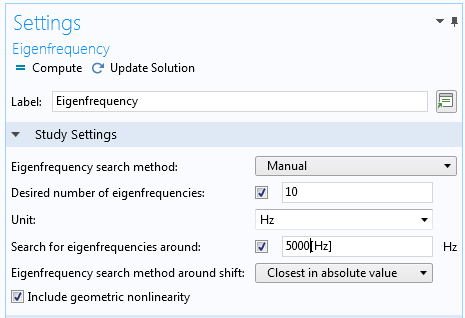The updated settings in the Eigenfrequency node.

Then, decrease the Relative tolerance in the settings for the Eigenvalue Solver node.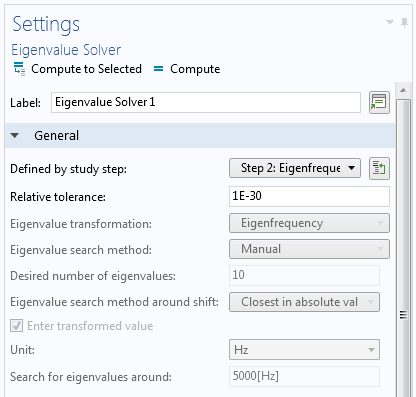The decreased Relative tolerance in the settings for the Eigenvalue Solver node.

Change only the parameters necessary for capturing the physics. For example, use the same mesh for all studies.

If you have reason to believe that the problem is ill-conditioned, as can be the case for a slender structure, select Iterative refinement in the settings for the Direct solver.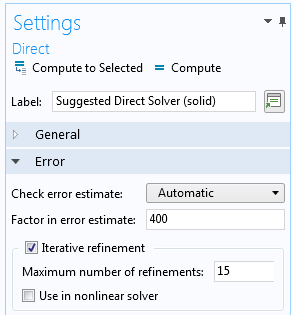The settings for the Direct solver, showing the option for Iterative refinement.

### A Theoretical Excursion into Handling Inelastic Strains Under Geometric Nonlinearity

In version 5.3 of COMSOL Multiphysics®, the method for how inelastic strains are handled under geometric nonlinearity has been changed. A multiplicative decomposition of deformation gradients is the current default, rather than the subtraction of strains that was used in previous versions. This is one key concept to understand why it is now possible to perform this type of analysis with a very high accuracy. Let’s look at a (somewhat artificial) case where the temperature increase is 3·104 K and there are no temperature dependencies in the material properties. This means that the stretches are

1+\epsilon_x = 1.3
1+\epsilon_y = 1.6
1+\epsilon_z = 1.9

resulting in the volume changing by a factor of 3.952.

You can then compare the results from the prestressed eigenfrequency analysis with a standard eigenfrequency analysis on a bigger beam with L = 13 mm; a = 1.6 mm; b = 0.95 mm; and lower density, scaled by a volume factor of 3.952, ρ = 253.036 kg/m3. This of course leads to large increases in the natural frequencies, as the heated object is much larger but with a lower density. The relative changes in frequency for the two approaches are shown in the following table.

Mode Type Thermal Expansion and
Prestressed Eigenfrequency
Larger Geometry and
Lower Density
First bending, z direction 2.2309 2.2308
First bending, y direction 1.8759 1.8759
First twisting 1.6702 1.6695
First axial 1.5292 1.5292

As can be seen above, the correspondence is in excellent agreement. There is a slight difference in the twisting mode, but that disappears with a refined mesh. Actually, refining the mesh shows that the best prediction is from the prestressed eigenfrequency analysis.

### Concluding Thoughts on Studying Eigenfrequencies That Change with Temperature

We have discussed how to accurately determine changes in eigenfrequencies caused by temperature changes with COMSOL Multiphysics, as well as the effects of stress softening, geometric changes, and the temperature dependence of material properties.

• Check out these blog posts on structural mechanics analysis:
• See updates to the Structural Mechanics Module on the Release Highlights page

#### Categories##### Ivar KJELBERG
May 28, 2017

Hei Henrik

Thanks for a very interesting blog. Specially you are “stressing” the delicate settings of the “Reference Temperature” and “Model Temperature” for the materials, and the too often “double effect” arriving when we add T dependence on material and do not check how it applies, in which frame we are.
I’m also noting the new approach to non linear geometry calculations, that might give us some surprises when comparing previous and new models after 5.3. We have many cases when we are comparing ppm level frequency changes due to second order T and sigma effects, for the watch industry. A mechanical “Chronomètre whatch” should have a precision of the order of a few ppm per day! This is high precision mechanics and material science.
We have noticed that if we cannot rely on the precision of the absolute frequency calculated due to mechanical tolerances of the parts we measure, but we have rather good agreements with the relative changes due to Temperature and Stress stiffening/softening that is happening some 100 thousand times, or more, per day in a mechanical watch.
Sincerely
IvarMarch 14, 2018

Hello Henrik

Thank you for the interesting blog. I am convinced with the shift in eigenfrequency with various conditions. Although, I am not sure on how COMSOL does the analysis.

In study 7 (in the application file) of the model discussed above, when I plot the store solution and the eigenmode, both of them do not have the same dimensions. Ideally, I expected the eigenmode to be displayed on the thermally expanded structure. So in the prestressed eigenfrequency analysis, does the eigenfrequency analysis use the deformed structure with thermal stresses as the initial condition? or does it use any other condition? Any help is much appreciated.

Thanks
Chaitanya##### Henrik Sönnerlind
March 15, 2018

Hi Chaitanya,

The problem is formulated on the initial geometry (‘material frame’ in our terminology). This can be seen as a transformation from the thermally expanded state. This approach has the drawback that mode shape, as a default, is plotted on the undeformed geometry.

You can create a mode plot with respect to the deformed geometry by manually superimposing the predeformation in the ‘Deformation’ node.

1. Use an expression for the deformation like

withsol(‘sol2’,u)+multiplier*u.

Here ‘sol2’ is assumed to be the solution containing the preload case. The ‘withsol’ operator can be used to pick up any solution explicitly, and thus allows a blending of results from for example different study steps.

2. Set the ‘Scale factor’ to 1 since you want true scale for the preload deformation.

3. The constant ‘multiplier’ will probably need to be adjusted for each mode in order to get a good visualization. The mode amplitudes can be made more uniform by setting ‘Scaling of eigenvectors’ to ‘Max’ in the ‘Eigenvalue’ Solver node.

Regards,
HenrikMarch 15, 2018

Thank you Henrik for your response.

Following up on my question, in study 2 (in the application file) I enabled and disabled the thermal expansion and I obtained the same set of eigenfrequencies, which is what I expected.

Similarly, in study 7, I set “beta” to be zero, ensuring modulus to be constant, in order to look at the frequency shift just due to thermal expansion. I disabled thermal expansion in both the study steps under the modify physics tree and I obtained the same set of frequencies as obtained in study 2. When I included thermal expansion in just the eigenfrequency step and not the stationary step, I expected the frequencies to be same as that from study 2. But, to my surprise, the frequencies are way off from what I expected.

Extending the above analysis, I enabled thermal expansion in the stationary step and disabled in the eigenfrequency step expecting the same results with the case where thermal expansion is enabled in both the steps. But it is not the case.

I am curious to know how modifying the physics tree effects the solutions. Looking forward to your response.

Thanks,
Chaitanya##### Henrik Sönnerlind
March 19, 2018

Hi Chaitanya,

If you think about how the equations as set up based on the weak form, the stiffness matrix is generated from the multiplication stress:test(strain). The stress is computed as C:elastic_strain where C is the elasticity tensor.

Without having a thermal expansion in the eigenfrequency step which matches the one used to compute the displacements of the preload step, the elastic strain (and thus the stress) at the linearization point will be way off. This causes a difference in the stiffness matrix and thus in the computed natural frequencies.

Regards,
HenrikMarch 26, 2018

Hello Henrik,

Thank you for your response. To thoroughly understand what was going on, I wanted to take a look at the stiffness matrices, K, for Cantilever without thermal expansion, Study 2 (sol2) and Cantilever with thermal stresses, Study 6 (sol9) using MATLAB live-link. The modulus is same for both the studies. And I obtained the exact same matrices. I expected a difference in the stiffness values as thermal expansion leads to change in the dimensions of the structure. Any insights into what is going on is much appreciated.

Thanks,
ChaitanyaMarch 27, 2018

Hello Henrik,

I also compared sol2 with sol15 (dT=30000K) and saw a maximum change in the order of 2.9802e-8

Best,
Chaitanya##### Henrik Sönnerlind
March 27, 2018

Hi Chaitanya,

I see significant differences (relative changes of the order 1e-4) when I check the stiffness matrices in the GUI (using an Assembly node and Derived Values->System Matrix) for sol2 and sol9. It seems like you have made a mistake in the export. My best guess is that you got the matrix without geometric nonlinearity or wrong linearization point.

Regards,
HenrikMarch 29, 2018

Hello Henrik,

If I understand it correctly, we are trying to find the eigenvalues of the equation Mx”+Kx=0. With temperature change the structure deforms thereby changing the K matrix to say K’. Now we solve the new equation to Mx”+K’x=0 to find the new set of eigenvalues. The new equation will account for deformation and the thermal stresses also taken into account into K’ while forming the stiffness matrix. Will there be any remeshing done between steps to account for change in shape?

Thanks,
ChaitanyaApril 3, 2018

Hello Henrik,

Can you tell me the equation COMSOL solves for in the second step (eigenfrequency) ? I want to understand how the geometric and pre-stress affects the system matrices (M & K).

Thank you for your time.

Chaitanya##### Henrik Sönnerlind
April 3, 2018

Hello Chaitanya,

The equations are always formulated in the undeformed configuration. Thus, the mass matrix is not affected. The stiffness matrix is changed because of the effects of initial stress and strain.

To actually compute the effects on the stiffness matrix requires an element-by-element evaluation, as is done in the assembly phase. Not all elements of the matrix scale in the same way.

To derive the formulation, you would have to set up the virtual work expressions in the the deformed configuration, and then change variables in the integrals in order to transform them to the original configuration.

Regards,
Henrik